# Dielectric Constant of Different Materials or Relative Permittivity

Dielectric Materials

A dielectric is an electrical insulator that can be polarized by an applied electric field. When a dielectric is placed in an electric field, electric charges do not flow through the material as they do in a conductor, but only slightly shift from their average equilibrium positions causing dielectric polarization. Because of dielectric polarization, positive charges are displaced toward the field and negative charges shift in the opposite direction. This creates an internal electric field which reduces the overall field within the dielectric itself.

While the term “insulator” implies low electrical conduction, “dielectric” is typically used to describe materials with a high polarizability. The latter is expressed by a number called the dielectric constant. The term insulator is generally used to indicate electrical obstruction while the term dielectric is used to indicate the energy storing capacity of the material (by means of polarization).

If the space between the plates of a capacitor is filled with a Dielectric, the capacitance of the capacitor will change compared to the situation in which there is vacuum between the plates. The change in the capacitance is caused by a change in the electric field between the plates. The electric field between the capacitor plates will induce dipole moments in the material between the plates. These induced dipole moments will reduce the electric field in the region between the plates.

A material in which the induced dipole moment is linearly proportional to the applied electric field is called a linear dielectric. For linear dielectric: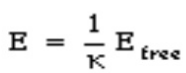Where K is called the dielectric constant. Since the final electric field E can never exceed the free electric field Efree, the dielectric constant k must be larger than 1.

The potential difference across a capacitor is proportional to the electric field between the plates.Since the presence of a dielectric reduces the strength of the electric field, it will also reduce the potential difference between the capacitor plates (if the total charge on the plates is kept constant):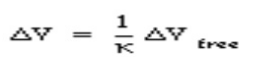The capacitance C of a system with a dielectric is inversely proportional to the potential difference between the plates, and is related to the capacitance C_free of a capacitor with no dielectric in the following manner: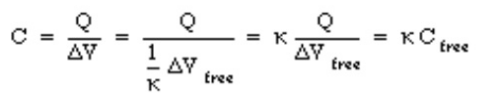Since k is larger than 1, the capacitance of a capacitor can be significantly increased by filling the space between the capacitor plates with a dielectric with a large k.

The electric field between the two capacitor plates is the vector sum of the fields generated by the charges on the capacitor plates and the field generated by the surface charges on the surface of the dielectric.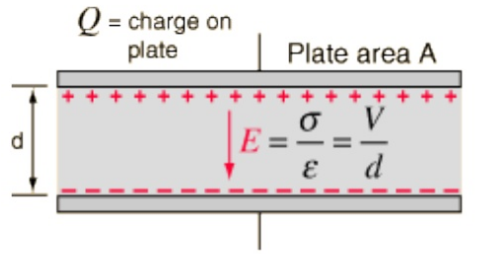The electric field between two large parallel plates is given by: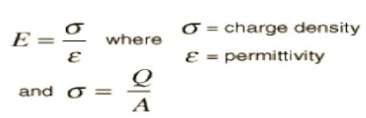The voltage difference between the two plates can be expressed in terms of the work done on a positive test charge q when it moves from the positive to the negative plate.It then follows from the definition of capacitance that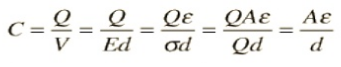### Dielectric Constant (Permitivity)

In Electromagnetism, permittivity is one of the fundamental material parameters, which affects the propagation of Electric Fields. Permittivity is typically denoted by the symbol ε. Absolute permittivity is the measure of the resistance that is encountered when forming an electric field in a medium. In other words, permittivity is a measure of how an electric field affects, and is affected by, a dielectric medium.

To understand permittivity, consider the Figure shown blow, in which two charged plates are separated, with equal and opposite charges on either side. Assume for the moment that between the plates, there is no material (vacuum).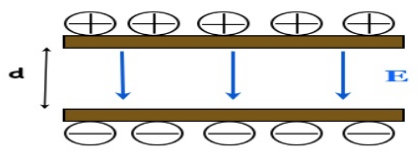As you can imagine, there will exist an Electric Field in Figure, directed downward (from the positive charge to the negative charge). Now, imagine that some material is placed between the plates. This material is no doubt made up of atoms which often form molecules, and as in the case of water, these molecules often look (electrically) like small dipoles (with a positive charge on one end and negative charge on the other end).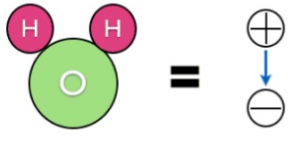In general, a material will be made up of some composition of molecules or atoms. These molecules will often have some sort of dipole movement. In the absence of an external electric field, these molecules will align randomly, as shown in Figure blow: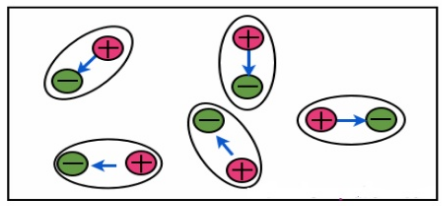Now, suppose this material is placed between the charged plates of above figure. The result is that the molecules will align themselves as shown in Figure blow: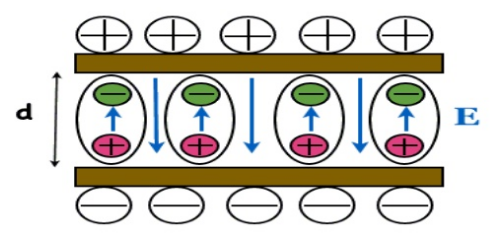The picture shows something very important – the electric field due to the dipole moment of the materials molecules opposes the external electric field E in last Figure. The result is that the net electric field is reduced within the material. Generally, permittivity will vary with frequency, temperature, and humidity. For many common materials this variation will be negligible. The permittivity is a measure of how much the molecules oppose the external E-field.

The E-field due to a single point charge of value q [C] at a distance R placed in vacuum is: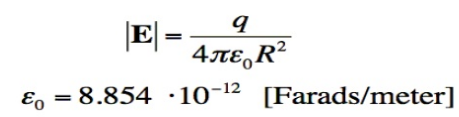In previous equation, is the permittivity of Free Space, which is measured in Farads/meter. This is the permittivity of a vacuum (no atoms present). In general, the Electric Field due to a point charge will be reduced due to the molecules within a material. The effect on the Electric Field is written in blow Equation: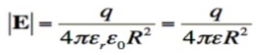The term εr is known as the relative permittivity or dielectric constant. Below table gives dielectric constant of different materials.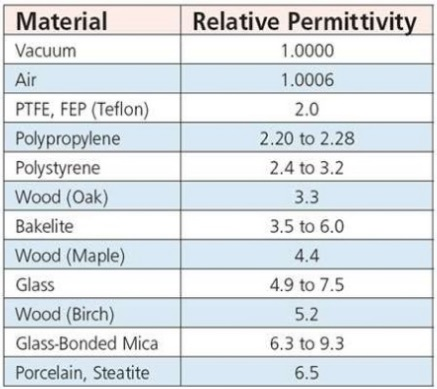error: Content is protected !!